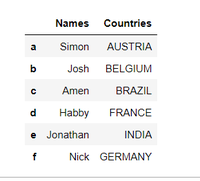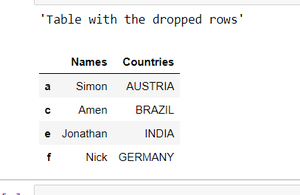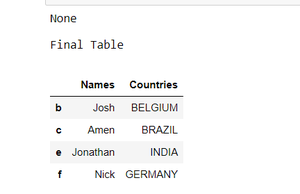GeeksforGeeks App
Open AppBrowser
Continue

# Drop a list of rows from a Pandas DataFrame

Let us see how to drop a list of rows in a Pandas DataFrame. We can do this using the Pandas drop() function. We will also pass inplace = True and axis=0 to denote row, as it makes the changes we make in the instance stored in that instance without doing any assignment.

## Python3

 `# import the module``import` `pandas as pd` `# creating a DataFrame``dictionary ``=` `{``'Names'``: [``'Simon'``, ``'Josh'``, ``'Amen'``, ``'Habby'``,``                        ``'Jonathan'``, ``'Nick'``],``              ``'Countries'``: [``'AUSTRIA'``, ``'BELGIUM'``, ``'BRAZIL'``,``                            ``'FRANCE'``, ``'INDIA'``, ``'GERMANY'``]}``table ``=` `pd.DataFrame(dictionary, columns``=``[``'Names'``, ``'Countries'``],``                     ``index``=``[``'a'``, ``'b'``, ``'c'``, ``'d'``, ``'e'``, ``'f'``])` `display(table)`

Output:### Drop a list of rows from a Pandas DataFrame using df.drop

In this example, we are simply dropping the row with the index 3.

## Python3

 `# drop 3rd row``display(``"Dropped 3rd row"``)``display(table.drop(``'c'``))`

### Delete rows from pandas without mentioning the index labels

Here, we are simply dropping rows 1 and 3 from the Dataframe table. At first, we dropped using the index value and after that, we use the row name to drop the row.

## Python3

 `# gives the table with the dropped rows``display(``"Table with the dropped rows"``)``display(table.drop(table.index[[``1``, ``3``]]))` `# You can also use index no. instead rows name``# display(table.drop(['a', 'd']))`

Output:### Drop a list of rows from a Pandas DataFrame using inplace

In this example, we are dropping the rows with and without inplace. Here, we use inplace=True which performs the drop operation in the same Dataframe, rather than creating a new Dataframe object during the drop operation.

## Python3

 `table ``=` `pd.DataFrame(dictionary, columns``=``[``'Names'``, ``'Countries'``],``                     ``index``=``[``'a'``, ``'b'``, ``'c'``, ``'d'``, ``'e'``, ``'f'``])` `# it gives none but it makes changes in the table``display(table.drop([``'a'``, ``'d'``], inplace``=``True``))` `# final table``print``(``"Final Table"``)``display(table)`

Output:### Drop Rows by Index Range in Pandas DataFrame

The range’s lower and upper limits are inclusive and exclusive, respectively. Accordingly, rows 0 and 1 will be removed, but row 2 won’t be.

## Python3

 `# table after removing range of rows from 0 to 2(not included)``table.drop(table.index[``0``:``2``], inplace``=``True``)` `display(table)`

Output:### Drop Rows with Conditions in Pandas

The Josh name from the Dataframe is dropped based on the condition that if df[‘Names’] == ‘Josh’], then drop that row. You can drop multiple rows with more conditions by following the same syntax.

## Python3

 `df ``=` `table``index_names ``=` `df[ df[``'Names'``] ``=``=` `'Josh'``].index``  ` `# drop these row indexes``# from dataFrame``df.drop(index_names, inplace ``=` `True``)` `display(table)`

Output:RECOMMENDED ARTICLES- How to drop rows in Pandas DataFrame by index labels?

My Personal Notes arrow_drop_up